# Analysis-using-tablesThe structural frame
The construction
The reinforcement I
The reinforcement II
Quantity/Cost estimation
Detailing drawings
Introduction >Wind and Seismic Forces >
Structural model and Analysis
Slabs
Seismic behavour of frames
Appendix A
Appendix B
Appendix C
Appendix D
Introduction >
Modelling slabsMaterials
To be continued >
Introduction

## Assumptions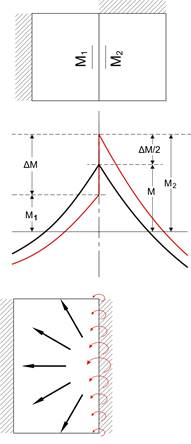A building usually comprises several, generally nonstandard, types of slabs (one-way, two-way etc.). In this case, each slab is analyzed separately by using tables. The moments M at the edge (border) of two slabs could be taken as the average of moments M1 (left slab moment) και M2 (right slab moment).

The difference ΔΜ=M2-M1 is redistributed in all directions of slabs, affecting less the other support moments and even lesser the span moments.

Special types of plates (inclined, of non-constant thickness etc.) could be solved as equivalent rectangular slabs of constant thickness.

## Application width of concentrated load on slab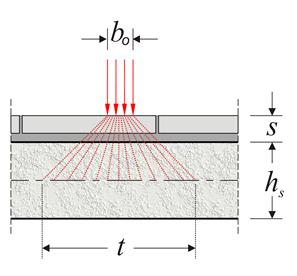The application width t of concentrated load P onto the middle level of the slab is expressed as:

t = b0 + 2×(s+hs/2) = b0 + 2×s + hs, where s is the covering thickness.

Thickness should not be taken into consideration, when the concentrated load is applied directly onto the concrete‘s surface, e.g. in the case of brick masonry.

## Distribution width of concentrated load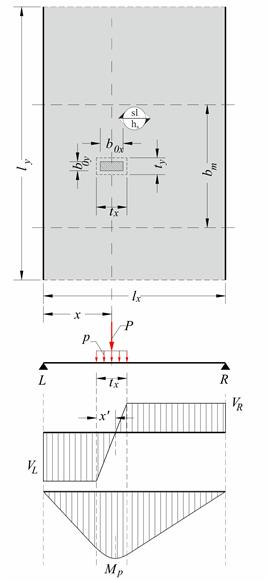The distribution width of the concentrated load is a function of the support types of the slab (fix, simple, free) and the distance x between the center of mass and the supports. The following table gives the distribution width of partial uniform load for every type of support.

## Distribution width of partial uniform load

 Simple slab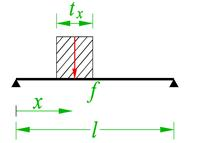bm,f = ty + 2.5×x×(1-x/l) tx,max = l, ty,max = 0.8l bm,vL = ty + 0.5×x tx,max = l, ty,max = 0.8l bm,vR = ty + 0.5×(l-x) tx,max = l, ty,max = 0.8l Example 1 bm,f=0.37+2.5×1.5×(1-0.375)=2.71 m bm,vL=0.37+0.5×1.5=1.12 m bm,vR=0.37+0.5×2.5=1.62 m Example 2 bm,f=0.37+2.5×2.0×(1-0.5)=2.87 m bm,vL=0.37+0.5×2.0=1.37 m bm,vR=0.37+0.5×2.0=1.37 m Simple with one end fixed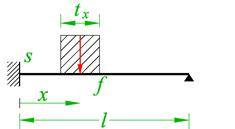bm,f = ty + 1.5×x×(1-x/l) tx,max = l, ty,max = 0.8l   bm,s = ty + 0.5×x×(2-x/l) tx,max = l, ty,max = 0.8l Left: για 0.2

## Example 1: simple slab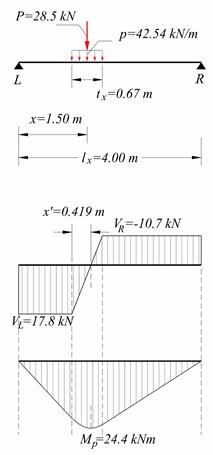Dimensions of slab lx=4.00 m, ly=5.00 m, width hs=170 mm. Subjected to covering load ge=1.0 kN/m2, live load q=5.0 kN/m2 and concentrated P (dead G=10.0 kN and live Q=10.0 kN), applied directly (s=0) onto the slab, with dimensions box=0.50 m, boy=0.20 m and distance of center of mass from left support x=1.50 m. L, R are pin supports. Calculate bending moment and shear force diagrams. The analysis with uniform loads is the same as in the example of §3.3.2 (vw=29.18 kN/m, mw=29.18 kNm/m). This design load is uniform along the entire length ly of the slab. The application width of the design concentrated load P is P=1.35×G+1.50×Q=1.35×10.0+1.50×10.0=28.5 kN tx=box+hs=0.50+0.17=0.67 m ty=boy+hs=0.20+0.17=0.37 m x/lx=1.5/4.0=0.375 The equivalent linear distributed load p in tx is p=P/tx=28.5kN/0.67m=42.5 kN/m The support shear forces are VL=Vx,L=p×tx×(lx-x)/lx=42.54×0.67×2.5/4.0=17.8 kN VR=Vx,R=Vx,L-p×tx=17.81-42.54×0.67=-10.7 kN The zero shear force point is located at x’=VL/p=17.81kN/(42.54kN/m)=0.419 m The maximum moment is Mp=AV =VL×(x-tx/2) + VL×x’/2=17.81×(1.5-0.67/2)+17.81 ×0.419/2=20.75+3.73=24.5 kNm The load widths are (previous table) tx,max=lx=4.0 m (>tx=0.67 m) και ty,max=0.8×lx=0.8×4.0=3.20 m (>ty=0.37 m) bm,f=ty+2.5×x×(1-x/l)=0.37+2.5×1.5×(1-0.375)=2.71 m και bm,vL=ty+0.5×x=0.37+0.5×1.5=1.12 m The additional design moment at a strip of width bm,f=2.71 m equal to mP=MP/bm,f=24.48kNm/2.71m=9.03 kNm/m while the total design moment is equal to mtot=mw+mP=29.18+9.03=38.21 kNm/m. The additional design shear force at a strip of width bm,vL=1.12 m is equal to vP=VL/bm,vL=17.81kN/1.12m=15.9 kN/m while the total design shear force is equal to vtot=vw+vP=29.2+15.9=45.1 kN/m.

## Example 2: simple slab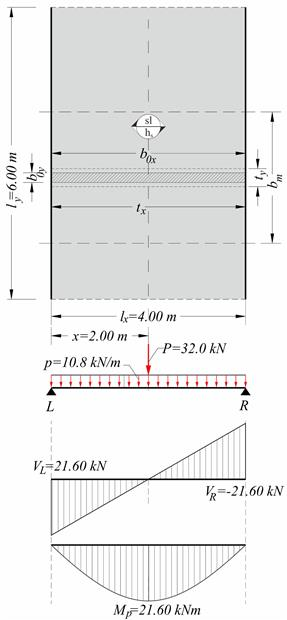In the previous slab, additional constant load g=8.0 kN/m is applied due to masonry from L to R (total load G=8.0kN/m·4.00m=32.0 kN) on width boy=0.20 m. Calculate bending moment and shear force diagrams. Solution: The analysis with uniform loads is the same as the previous example. The load strip dimensions are box=4.00 m and boy=0.20 m. The application width of the design concentrated load p is p=γg·g=1.35·8.0kN/m=10.8 kN/m tx=min(box+hs, lx)=min(4.00+0.17, 4.00)=4.00 m ty=boy+hs=0.20+0.17=0.37 m x=l/2=4.00/2=2.00 m x/l=2.00/4.00=0.50 The support shear forces are VL=VR=p·lx/2=10.8·4.00/2=21.6 kN Maximum mome Mp=p·l2x/8=10.8·4.002/8=21.6 kNm. The distribution load widths are (previous table) tx=tx,max=lx=4.00 m, ty,max=0.8·lx=0.8·4.00=3.20 m(>ty=0.37 m) bm,f=ty+2.5·x·(1-x/l)=0.37+2.5·2.00·(1-0.50) =2.87 m bm,vL=bm,vR=ty+0.5·x=0.37+0.5·2.00=1.37 m The additional design moment at a strip of width bm,f=2.87 m is equal to mp=Mp/bm,f=21.60kNm/2.87m=7.5 kNm/m while the total design moment is equal to mtot=mw+mP=29.2+7.5=36.7 kNm/m. The additional design shear force at a strip of width bm,vL=1.37 m is equal to vP=VL/bm,v=21.6kN/1.37m=15.8 kN/m while the total design shear force is equal to vtot=vw+vP=29.2+15.8= =45.0 kN/m.

## Example 3: Two-way slab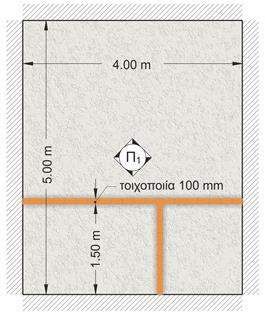In the case of two-way slab, concentrated or linear loads are distributed all over the slab as equivalent uniform load. This load is added to other uniform loads of the slab.

Example: The equivalent uniform linear load of masonries of the figure (e.g. the weight of masonry minus the weight of the door), along directions of 4.00 and 1.50 m are equal to 5.0 kN/m and 6.0 kN/m, respectively. Calculate the equivalent uniform load of the masonries distributed in the entire surface of the slab.

4.00m×5.0kN/m+1.50m×6.0kN/m=29.0 kN

29.0kN/(4.00m×5.00m)=1.45 kN/m2

## Support moments of continuous slabs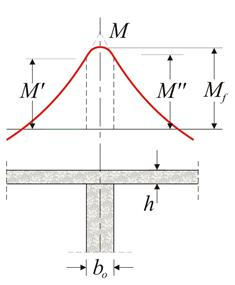In (structurally) pinned supports of slab, e.g. as in precast slabs, the moment is equal to: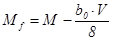, where V is the design support reaction.

In monolithic supports of continuous slabs, as usually constructed in practice, the moments Μ', Μ'' at the two sides are: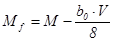,(Μ, V in absolute values) or approximately M’=M’’=0.90×M

The moments Μ', Μ'' at the two sides should be greater than 0.65 of the principal moments Mo,l and Mo,r of the corresponding slab with fully fixed ends.

## Principal moments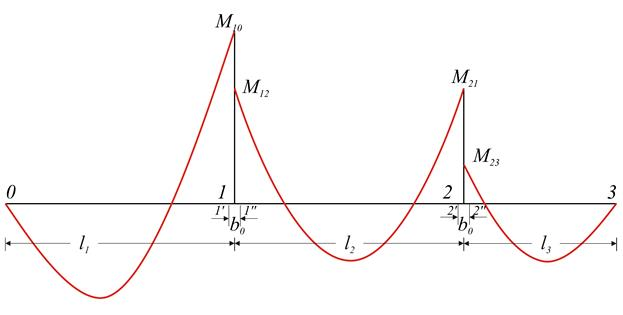The principal moments, under uniform loads, are equal to
M
10=p1l12/8, M12=M21=p2l22/12, M23= p3l32/8.

## Redistribution of stress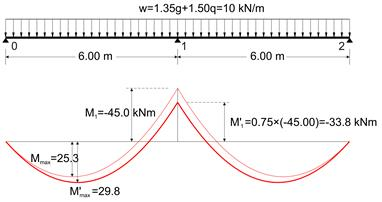In continuous slabs, support moments are allowed to be decreased [EC2, §5.5(4)] up to 30% when reinforcing steel of B or C category is used and up to 20% when reinforcing steel of A category is used, provided the increment of the respective span moments.

This redistribution allows the required thickness due to bending to be decreased, while the simultaneous increment of the reinforcement is relatively small.

Redistribution of 25 % of total moment example:

For support moment Μ1=-45.0 kNm, shear force is V0=10.0×6.00/2-45.0/6.00=22.5 kN and zero shear point (maximum moment point, see §3.3.3, sub note 10) is positioned at x=22.5/10.0=2.25 m. The maximum span moment at this point is Mmax=V0×x/2=22.5×2.25/2=25.3 kNm.

Reducing support moment Μ1 by 25%, we have:

M’1=-45.0×0.75=-33.8 kNm

V’0=10.0×6.00/2-33.8/6.00=24.4 kN

x=24.4/10.0=2.44 m

M’max=V’0×x/2=24.4×2.44/2=29.8 kNm

Note that the span moment is increased by (29.8-25.3)/25.3=18%.

## Contribution of pinned slab supports

Elastic analysis and use of table for two-way slabs, assume that corners are prevented from lift-ing and that diagonal twisting moments at those corners are carried by longitudinal reinforcement grid in upper and lower fibers. These two structural conditions are satisfied in practice, since rein-forcement of slabs interlace with stirrups and longitudinal reinforcement of beams, thus prevent-ing the lift of corners and creating a reinforcement grid in upper and lower fibers of the corners. Where (mandatory) construction standards (see volume Α’ §3.5) are not fully satisfied, although the equilibrium conditions will be met for the slabs, the span moments should increase by 5% - 33%.